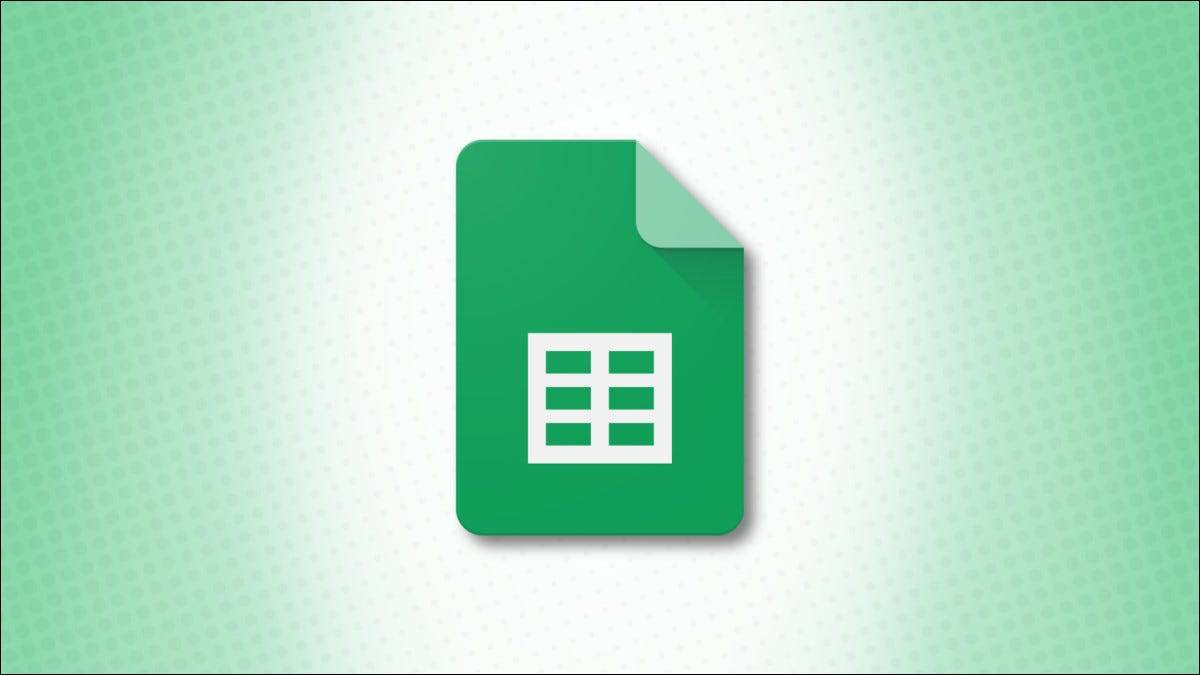## 计算单元格中的字符

Google 表格中的LEN 函数的工作方式与 Microsoft Excel 中的一样。它使用简单的公式为您提供单元格中的字符数

`=LEN(A1)`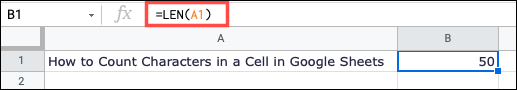`=LEN("明天又是一天")`## 计算一系列单元格中的字符

SUMPRODUCT 函数计算数组或单元格区域的总和。它的语法是`SUMPRODUCT(array1, array2, ...)`只需要第一个参数的地方。

`=SUMPRODUCT(LEN(A1:A5))`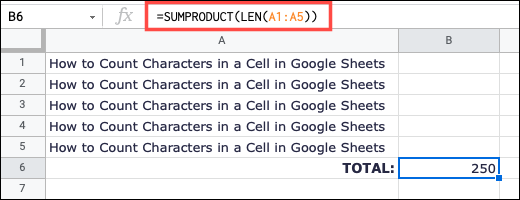## 计算没有额外空格的字符`=LEN（修剪（A10））`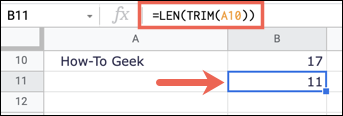## 计算单元格中特定字符的实例

SUBSTITUTE 函数通常用于替换单元格中的文本，它的语法是`SUBSTITUTE(current_text, find, new_text, occurrence)`需要前三个参数的地方。

`=LEN(A1)-LEN(替代(A1,"C",""))`

• `SUBSTITUTE(A1,"C","")`将每个 C 替换为引号中的内容，这什么都不是。
• `LEN(SUBSTITUTE(A1,"C","")`计算不是（替换的）字母 C 的字符数。
• `LEN(A1)`计算单元格 A1 中的字符。`=LEN(A1)-LEN(替换(UPPER(A1),"C",""))`
`=LEN(A1)-LEN(替代(LOWER(A1),"c",""))`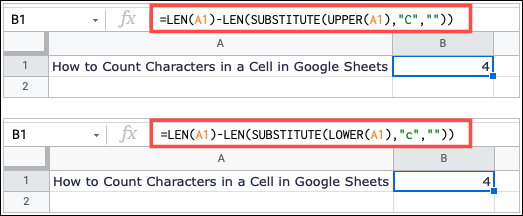94606652314人已关注

### 表示一下诚意

#### 支付宝扫一扫打赏#### 微信扫一扫打赏